::  Laboratories of Electronics & Communication Engineering Department  ::

• Basic Electronics Engg
• Circuits & Network
• Solid State Devices
• Signals and Systems
• Analog Electronics Circuits
• Electromagnetic Wave and Transmission Lines
• Digital Electronic & Integrated Circuits
• Analog Communication
• Control System
• Digital Communication
• Digital Signal Processing
• VLSI Design
• RF & Microwave Engineering
• Design
• Project
• Basic Electronics Engineering Laboratory (ES191/ES291)This is a lab for 1year B-Tech students which is divided into two part.Part-1 focuses on first semester as ES-191 and Part-2 focuses on second semester as ES-291

ES-191

List of Experiments:
1. Familiarisation with passive and active electronic components such as Resistors, Inductors, Capacitors, Diodes, Transistors (BJT) and electronic equipment like DC power supplies, multimeters etc.
2. Familiarisation with measuring and testing equipment like CRO, Signal generators etc.
3. Study of I-V characteristics of Junction diodes.
4. Study of I-V characteristics of Zener diodes.
5. Study of Half and Full wave rectifiers with Regulation and Ripple factors.
6. Study of I-V characteristics of BJTs. Circuit Theory & Network Lab.

ES-291

List of Experiments:
1. Study of I-V characteristics of Field Effect Transistors.
2. Determination of input-offset voltage, input bias current and Slew rate of OPAMPs.
3. Determination of Common-mode Rejection ratio, Bandwidth and Off-set null of OPAMPs.
4. Study of OPAMP circuits: Inverting and Non-inverting amplifiers, Adders, Integrators and Differentiators.
5. Study of Logic Gates and realization of Boolean functions using Logic Gates.
6. Study of Characteristic curves for CB, CE and CC mode transistors.

• Circuits & Network Lab oratory(EC391)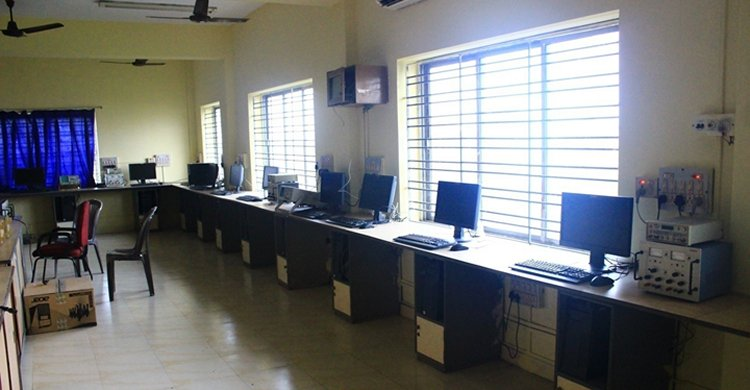This lab is for 2nd year B-tech students of Electronics and Communication Engineering.

List of Experiments:
1. Characteristics of Series & Parallel Resonant circuits
2. Verification of Network Theorems
3. Transient Response in R-L & R-C Networks; simulation / hardware
4. Transient Response in RLC Series & Parallel Circuits & Networks; simulation / hardware
5. Determination of Impedance (Z), and Admittance (Y) parameters of Two-port networks
6. Generation of periodic, exponential, sinusoidal, damped sinusoidal, step, impulse, and ramp signals using MATLAB
7. Representation of Poles and Zeros in s-plane, determination of partial fraction expansion in s-domain and cascade connection of second-order systems using MATLAB
8. Determination of Laplace Transform, different time domain functions, and Inverse Laplace Transformation using MATLAB.

• Solid State Devices Laboratory(EC392)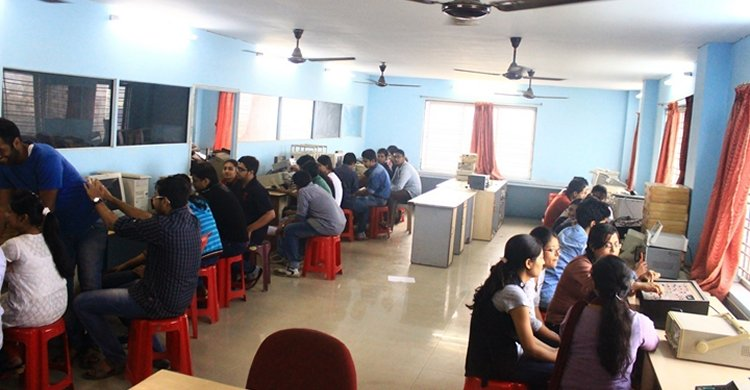This lab is for 2nd year B-tech students of Electronics and Communication Engineering.

List of experiments:
Module 1:
1. Study input characteristics of BJT in common-emitter configuration.
2. Study output characteristics of BJT in common-emitter configuration for different base currents and hence determine hybrid parameters.
3. Study output characteristics of BJT in common-emitter configuration and find performance parameters (Voltage Gain, Current Gain, Input Impedance, Output Impedance).
4. Study the variation of small-signal voltage gain with frequency of a common-emitter RC coupled amplifier.
5. Study of drain characteristics and transfer characteristics of a JFET and hence determine the FET parameters (drain resistance, trans conductance& amplification factor).
6. Study the variation of small-signal voltage gain with frequency of a JFET.

Module 2:
Perform any two experiments
1. Study of C-V characteristics of a Varactor diode by appropriate software.
2. Study of C-V characteristics of a MOS structure by appropriate software.
3. Study of drain characteristics and transfer characteristics of a MOSFET and hence determine the FET parameters (drain Resistance, Trans conductance & amplification factor).

• Signals and Systems Laboratory (EC393)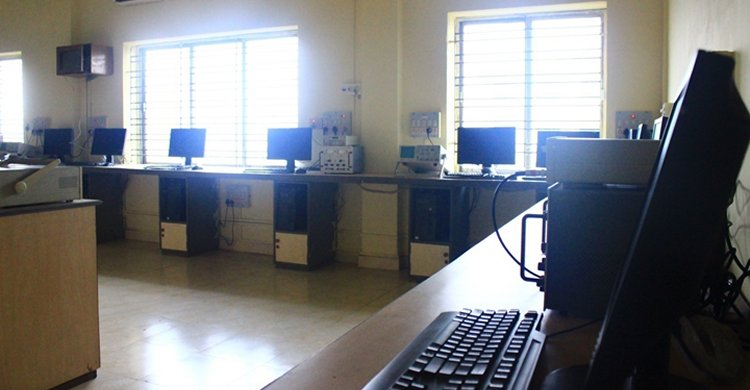This lab is for 2nd year B-tech students of Electronics and Communication Engineering.

List of experiments:
1. To study Z- transform of: a) Sinusoidal signals b) Step functions
2. To compare Fourier and Laplace transformations of a signal.
3. To study convolution theorem in time and frequency domain.
4. To Study Signal Synthesis via sum of harmonics.
5. To study LPF &HPF, band pass and reject filters using RC circuits.
6. To demonstrate how analog signals are sampled and how different sampling rates affect the outputs.
7. To study sampling theorem for low pass signals and band pass signals .
8. To determine the components of: a) Square wave b) Clipped sine wave.

• Analog Electronics Circuits Laboratory (EC394)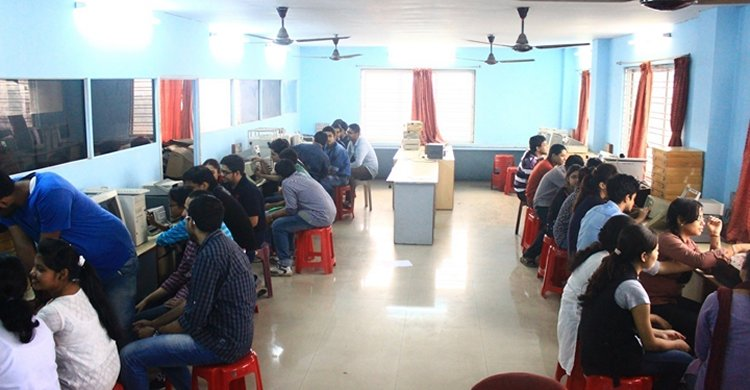This lab is for 2nd year B-tech students of Electronics and Communication Engineering.

List of experiments:
1. Study of Diode as clipper & clamper
2. Study of Zener diode as a voltage regulator
3. Study of ripple and regulation characteristics of full wave rectifier without and with capacitor filter
4. Study of characteristics curves of B.J.T &F.E.T .
5. Design a two-stage R-C coupled amplifier & study of itï¿½s gain & Bandwidth.
6. Study of class A & class B power amplifiers.
7. Study of class C & Push-Pull amplifiers.
8. Realization of current mirror & level shifter circuit using Operational Amplifiers.
9. Study of timer circuit using NE555 & configuration for monostable & astablemultivibrator.
10. Design a Bistablemultivibrator using NE 555.
11. Study of Switched Mode Power Supply & construction of a linear voltage regulator using regulator IC chip.
12. Design a simple function generator using IC.
13. Realization of a V-to-I & I-to-V converter using Op-Amps.
14. Realization of a Phase Locked Loop using Voltage Controlled Oscillator (VCO).
15. Study of D.A.C & A.D.C.

• Electromagnetic Wave and Transmission Lines Laboratory (EC491)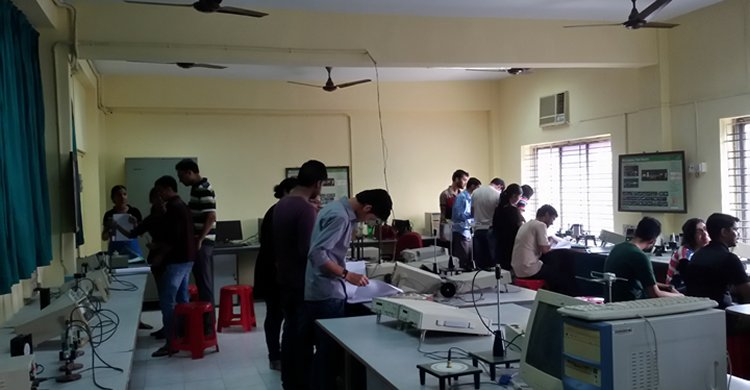This lab is for 2nd year B-tech students of Electronics and Communication Engineering.

List of experiments:
Module I:
1. Plotting of Standing Wave Pattern along a transmission line when the line is open-circuited, short-circuited and terminated by a resistive load at the load end.
2. Input Impedance of a terminated coaxial line using shift in minimal technique.
3. Study of Smith chart on Matlab platform.
4. Simulation study of Smith chart - Single and double stub matching.

Module II:
5. Radiation Pattern of dipole antenna.
6. Radiation Pattern of a folded-dipole antenna.
7. Radiation pattern of a 3-element Yagi-Uda Antenna.
8. Beam width, gain and radiation pattern of a 3-element, 5-element and 7-element. Yagi-Uda antenna - Comparative study.
9. Radiation pattern, Gain, Directivity of a Pyramidal Horn Antenna.
10. Study of Spectrum Analyzer Digital Electronic & Integrated Circuits Lab

• Digital Electronic & Integrated Circuits Laboratory(EC492)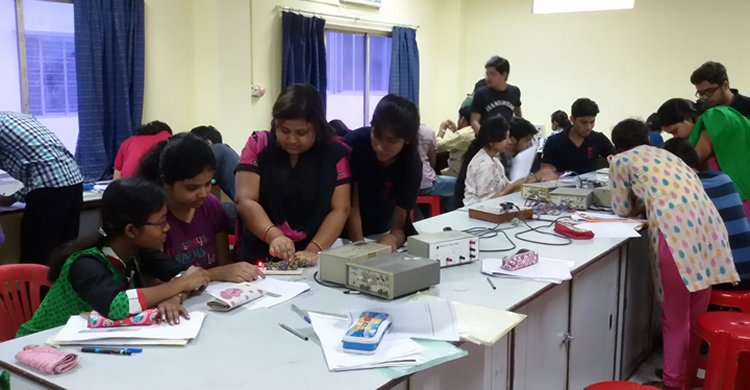This lab is for 2nd year B-tech students of Electronics and Communication Engineering.

List of experiments:
2. Code conversion circuits- BCD to Excess-3 and vice-versa.
3 Four-bit parity generator and comparator circuits.
4. Construction of simple Decoder and Multiplexer circuits using logic gates.
5. Design of combinational circuit for BCD to decimal conversion to drive 7-segment display using Multiplexer.
6. Construction of simple arithmetic circuits-Adder, Subtractor.
7. Realization of RS-JK and D flip-flops using Universal logic gates.
8. Realization of Universal Register using JK flip-flops and logic gates.
9. Realization of Universal Register using multiplexer and flip-flops.
11. Realization of Asynchronous Up/Down counter.
12. Realization of Synchronous Up/Down counter.
13. Design of Sequential Counter with irregular sequences.
14. Realization of Ring counter and Johnsonï¿½s counter.

• Analog Communication Laboratory (EC591)This lab is for 3rd year B-tech students of Electronics and Communication Engineering.

List of experiments:
1. Measurement of modulation index of an AM signal.
2. Measurement of output power with varying modulation index an AM signal(for both DSB- & SSB).
3. Measurement of distortion of the demodulated output with varying modulation index of an AM signal (for both DSB- SC & SSB).
4. Measurement of power of different frequency components of a frequency modulated signal & the measurement of the bandwidth.
5. Design a PLL using VCO & to measure the lock frequency.
6. Design a FM demodulator using PLL.
7. Measurement of SNR of a RF amplifier.
8. Measurement of selectivity, sensitivity, fidelity of a superhetrodyne receiver.
9. Study of waveforms of various functional points (output of RF,IF& video) of a B/W TV receiver.
10. Study of the vertical & horizontal sweep of the time base unit of a B/W TV.
11. Innovative experiments.

• Control System Laboratory (EC583)This lab is for 3rd year B-tech students of Electronics and Communication Engineering.

List of experiments:
1. Familiarization with MATLAB Control System tool Box, MATLAB- SIMULINK tool box & pSPICE.
2. Determination of step response for 1st order & 2nd order system with amity feedback on CRO & calculation of control system specifications for variations of system design.
3. Simulation of step response & impulse response for Type-I & Type-II system with unity feedback using MATLAB &pSPICE.
4. Determination of root locus, Bode-plot, Nyquist Plot, using MATLAB control system toolbox for a given 2nd order transfer function & determination of different control system specifications.
5. Determination of PI, PD, and PID controller action on 1st order simulated process
6. Determination of approximate transfer function experimentally using Bode Plot.
7. Evaluation of steady-state error, setting time, percentage peak overshoots, gain margin, phase margin with addition of lead compensator in forward path transfer functions using MATLAB &pSPICE.
8. Study of position control system using servomotor.
9. Design and hardware implementation of a temperature controller using microprocessor/microcontroller.

• Digital Communication Laboratory(EC691)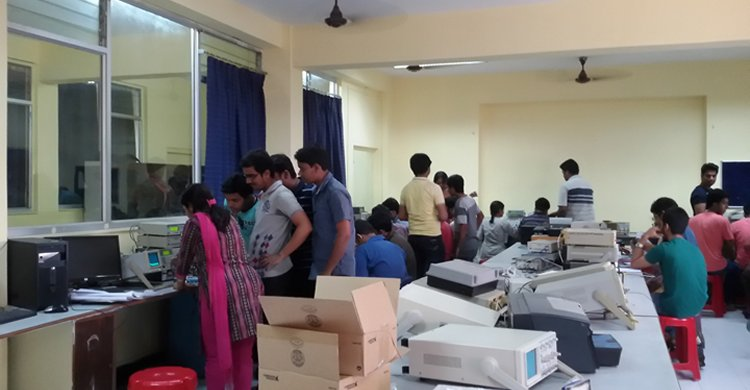This lab is for 3rd year B-tech students of Electronics and Communication Engineering.

List of experiments:
1. Design, implementation and study of all the properties of 7-length and 15-length pn sequences using shift register.
2. Study of PAM and demodulation.
3. Study of PCM and demodulation.
4. Study of line coders: polar/unipolar/bipolar NRZ, RZ and Manchester.
5. Study of delta modulator and demodulator.
6. Study of adaptive delta modulator and demodulator.
7. Study of BPSK modulator and demodulator.
8. Study of BFSK modulator and demodulator.
9. Study of ASK modulator and demodulator.
10. Study of QPSK modulator and demodulator.
11. Simulation study of probability of symbol error for BPSK modulation.
12. Simulation study of probability of symbol error for BFSK modulation.

• Digital Signal Processing Laboratory (EC692)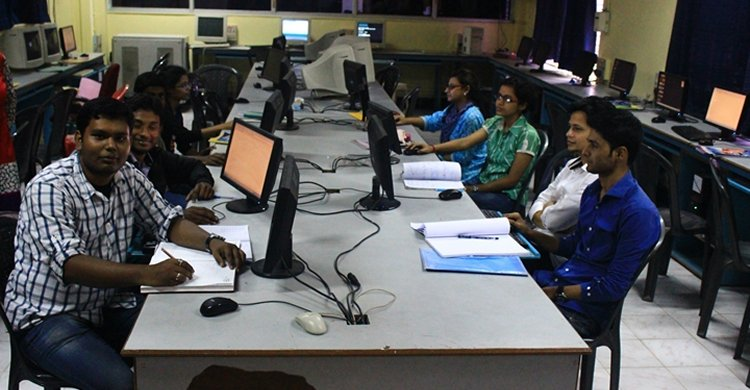This lab is for 3rd year B-tech students of Electronics and Communication Engineering.

List of experiments:
Simulation Laboratory using standard Simulator:
1. Sampled sinusoidal signal, various sequences and different arithmetic operations.
2. Convolution of two sequences using graphical methods and using commands- verification of the properties of Convolution
3. Z-transform of various sequences - verification of the properties of Z-transform.
4. Twiddle factors - verification of the properties.
5. DFTs / IDFTs using matrix multiplication and also using commands.
6. Circular convolution of two sequences using graphical methods and using commands, differentiation between linear and circular convolutions.
7. Verifications of the different algorithms associated with filtering of long data sequences and Overlap - add and Overlap- save methods.
8. Butterworth filter design with different set of parameters.
9. FIR filter design using rectangular, Hamming and Blackman windows.

Hardware Laboratory using either 5416 or 6713 Processor and Xilinx FPGA:
1. Writing & execution of small programs related to arithmetic operations and convolution using Assembly Language of TMS320C5416/6713 Processor, study of MAC instruction.
3. Mapping of some DSP algorithms onto FPGA.

• VLSI Design Laboratory( EC792)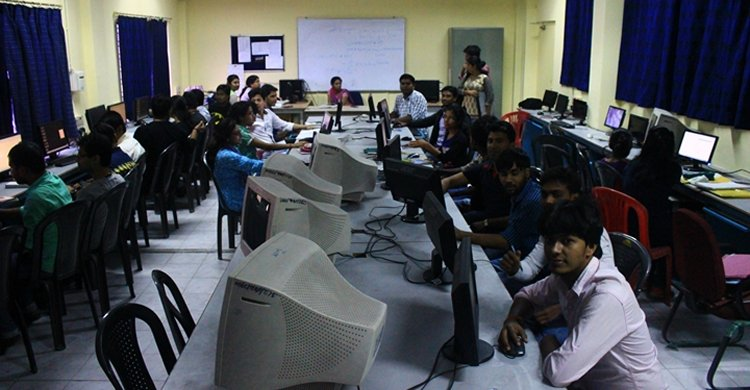This lab is for 4th year B-tech students of Electronics and Communication Engineering.

List of experiments:
1. Familiarity with Spice simulation tool .
2. Spice Simulation of Inverter , NAND , NOR Gates.
3 Familiarity with EDA tools for VLSI design /FPGA based system design.
4. Layouts, Transistors and tools,.
5. Standers cell Design.
6. Design of CMOS XOR/XNOR Gates.
7. Design of CMOS Full adder.
8. Design of CMOS Flip flops ( R-S ,D , J-K).
10. Design of 8 bit synchronous Counter.
11. Design of 8 bit bi-directional register with tri-stated input/output bus. 12. Design of a 12 bit CPU with few instructions and implementation and validation on FPGA.

• RF & Microwave Engineering Laboratory(EC793A)This lab is for 4th year B-tech students of Electronics and Communication Engineering.

List of experiments:
1. Determination of phase and group velocities in a waveguide carrying TE10 Wave from Dispersion diagram [w-ï¿½ Plot].
2. Measurement of unknown impedance using shift in minima technique using a waveguide test bench/ Measurement of the susceptance of an inductive and or a capacitive window using shift in minima technique using a waveguide test bench
3. Study of the characteristics of a Reflex Klystron oscillator
4. Study of Gunn-oscillator Characteristics using X-band waveguide test bench.
5. Measurement of coupling factor, Directivity, Insertion loss and Isolation of a Directional coupler using X-band waveguide test bench set up.
6. Scattering matrix of a magic tee / E-plane tee / H-plane tee using waveguide test bench at X-band.
7. Experimental/Simulation Study of filter (LPF, HPF,BPF) response.
8. Measuring of dielectric constant of a material using waveguide test bench at X-band.

• Design Laboratory (EC881)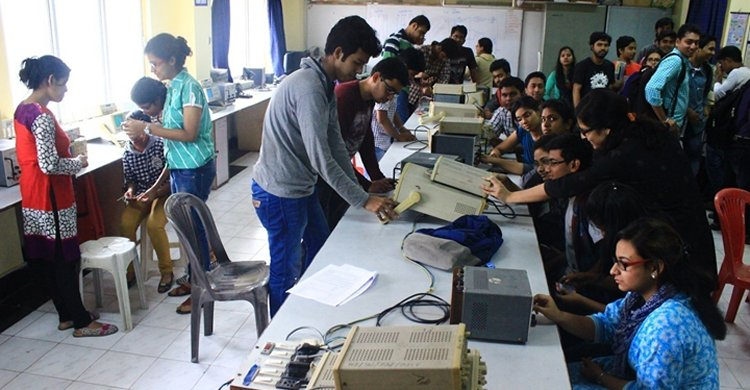This lab is for 4th year B-tech students of Electronics and Communication Engineering.

List of experiments:
A. DISCRETE ANALOG CIRCUITS
1. Rectifiers.
(To design a rectifier for a given average output dc voltage and a given load resistance, compare between the theoretical values of Vdc, Vrms, RF, HD, output regulation, transformer utility factor etc. with the measured values, and thus comprehend the relevance/effect of these various parameters.)
2. DC power supplies regulation and protection circuits.
(To learn designing a series transistor based output regulation circuit, an output current limiting circuit, fold back circuit needed for a given output parameters.)
3. Single stage audio frequency voltage amplifier with BJT for a given Av, Zin and Zout and maximum symmetrical output swing.
(To learn basic design principles, different methods of biasing, bias stability, selection of transistor from data manuals and effect of ac coupling on bandwidth.)
4. Single stage audio frequency emitter follower with JFET for a given Av, Zin and Zout and maximum symmetrical output swing.
(To learn the design principles and applications of an emitter follower.)
5. Complimentary symmetry power amplifier with pre amplifier, if necessary, for a given out put power to a given load with single ended power supply.
(To learn the distinction of a power amplifier over and above a voltage or current amplifier, its design principles, issues like,efficiency, cross over distortion etc.)
6. RC phase shift Oscillator , Wien Bridge oscillator, Hartley and Colpitt oscillator
( To learn the design of oscillators and measuring the frequency and amplitude of oscillations)

B. OPAMP BASED ANALOG CIRCUITS
1. Inverting and non-inverting amplifier of given dc gain, input impedance and output impedance.
(To learn the basic design, inter relation between the dc gain and input/output impedances, offset balance and the relation between feedback and GBW.)
(To learn the basic design and function of a multi input adder/subtractor (with ac and dc inputs present simultaneously).
3. Comparator/voltage level detector for a given upper threshold level and a given lower threshold level with facility of independent adjustment of hysteresis and center point.
(To learn the design and the technique of independent adjustment of both hysteresis and center point.)
4. Active filters: LP, BP, HP, 1st order, 2nd order.
(To learn the design of a filter and itï¿½s inherent phase shifting characteristics.)
5. 555 based monostable and astable of duty cycle below and above 50%.
(To learn designing 555 based timer circuits.)

C.DIGITAL LOGIC CIRCUITS
1. Design and implement a BCD to 7-segment decoder with basic and universal gates.
(To understand clearly the method of writing a truth table, use of K-map, simplifying a logic function and optimum design with Minimum number of ICs and inputs.).

• Project Laboratory (EC 881/EC882)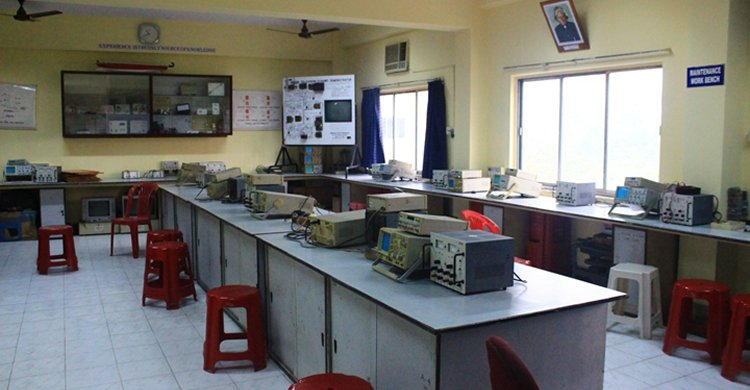This is a lab mainly for 4th year ECE students for doing their hardware based project works.